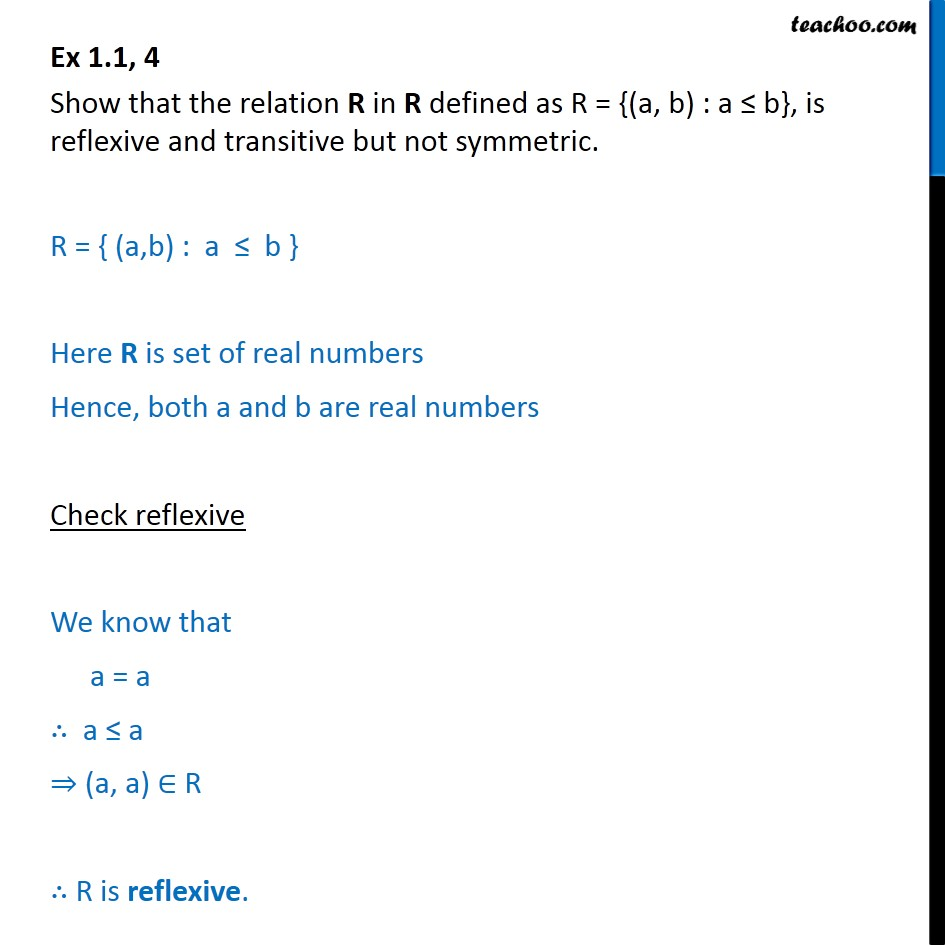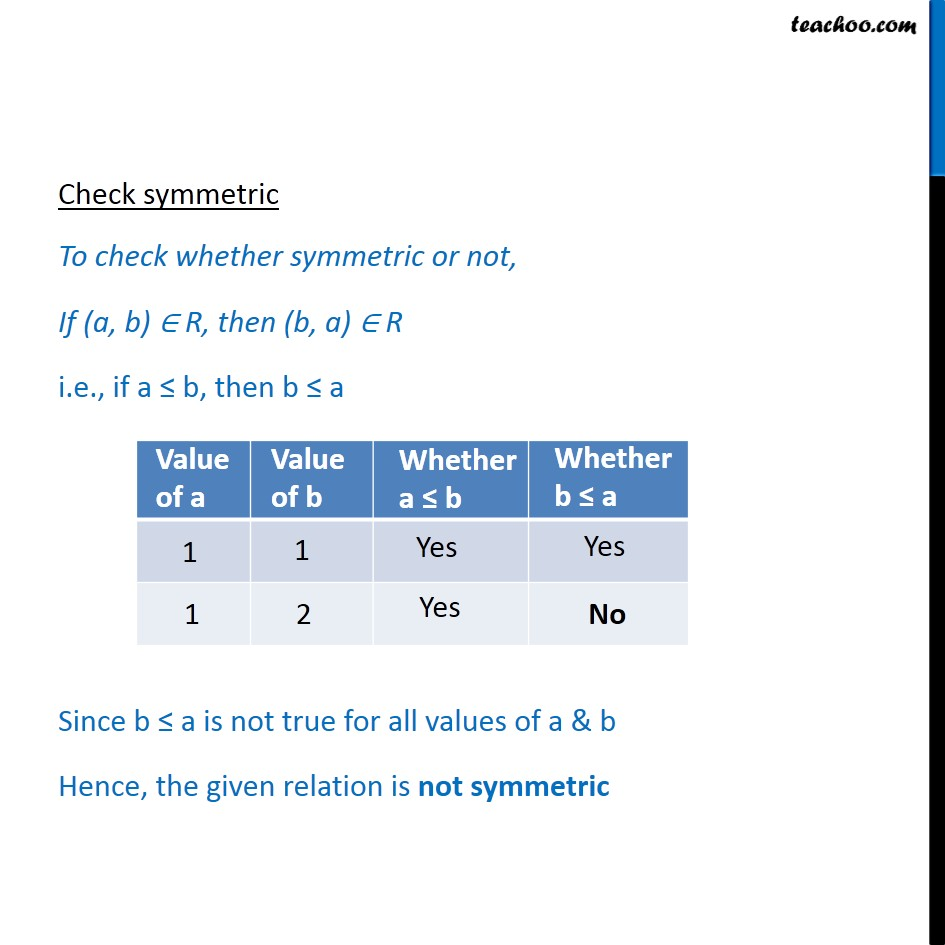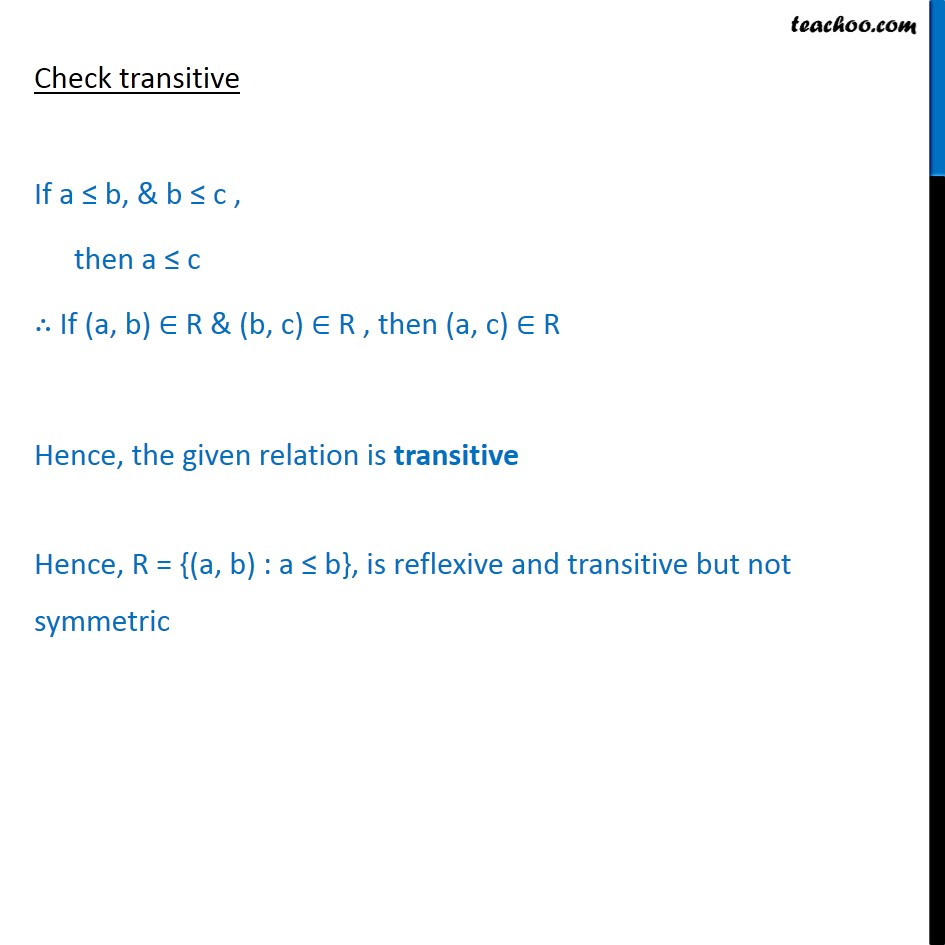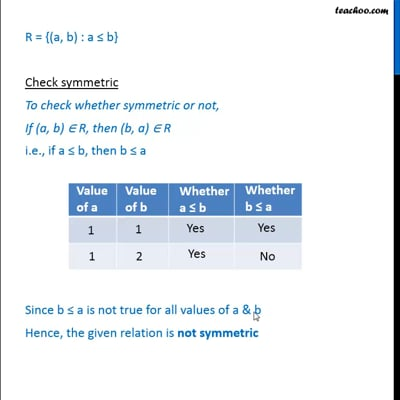Ex 1.1

Chapter 1 Class 12 Relation and Functions
Serial order wiseThis video is only available for Teachoo black users

Introducing your new favourite teacher - Teachoo Black, at only ₹83 per month

### Transcript

Ex 1.1, 4 Show that the relation R in R defined as R = {(a, b) : a b}, is reflexive and transitive but not symmetric. R = { (a,b) : a b } Here R is set of real numbers Hence, both a and b are real numbers Check reflexive We know that a = a a a (a, a) R R is reflexive. Check symmetric To check whether symmetric or not, If (a, b) R, then (b, a) R i.e., if a b, then b a Since b a is not true for all values of a & b Hence, the given relation is not symmetric Check transitive If a b, & b c , then a c If (a, b) R & (b, c) R , then (a, c) R Hence, the given relation is transitive Hence, R = {(a, b) : a b}, is reflexive and transitive but not symmetric